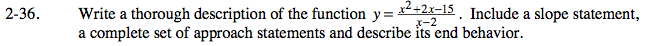### Home > CALC > Chapter 2 > Lesson 2.1.3 > Problem2-36

2-36.Factor the numerator and simplify the ratio to determine the location of holes and/or vertical asymptotes.

No holes (the ratio does not simplify). Vertical asymptote at x = 2.

Evaluate values close to x = 2 (from the left and from the right) to determine if the function approaches its asymptote in an increasing or decreasing manner.

$y(2^{-})\approx y(1.9)=\frac{(1.9+5)(1.9-3)}{(1.9-2)}=\frac{(5.9)(-1.1)}{(-0.1)}=64.9 \ \ \ \text{going up from the left}$

This will help you with the approach statements for x → 2+ and x → 2.

Use long division to determine the end behavior.

This will also help you write the approach statements for x → − ∞ and x → ∞.

Use the graph to write your approach statements and your slope statement.

Use the eTool below to view the graph for each step.
Click on the link to the right to view the full version of the eTool. Calc 2-36 HW eTool